# Summerjob

Albert, Beny, Cyril and Dan worked on summerjob. From the total revenue Albert received 2/5, Beny 1/6 , 3/10 Cyril and Dan the rest which is 324 euros.

How many euros earned every one of them?

A =  972 Eur
B =  405 Eur
C =  729 Eur
D =  324 Eur

### Step-by-step explanation:

A = 2/5 x
B = 1/6 x
C = 3/10 x
D = 324
D = x-A-B-C

A = 2/5•x
B = 1/6•x
C = 3/10•x
D = 324
D = x-A-B-C

5A-2x = 0
6B-x = 0
10C-3x = 0
D = 324
A+B+C+D-x = 0

A = 972
B = 405
C = 729
D = 324
x = 2430

Our linear equations calculator calculates it.Did you find an error or inaccuracy? Feel free to write us. Thank you!Tips to related online calculators
Need help to calculate sum, simplify or multiply fractions? Try our fraction calculator.
Do you have a linear equation or system of equations and looking for its solution? Or do you have a quadratic equation?

## Related math problems and questions:

• StudentsStudents Aleš, Bohouš, Cyril, and Dušan were on a brigade. They divided the total revenue as follows: Aleš received two-fifths of the revenue, Bohouš received one-sixth of the revenue, Cyril received three-tenths of the revenue, and Dušan received the res
• Email hoard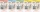Kendrick received 24 fewer e-mails on Friday than he did on Thursday. If he received a total of 126 e-mails on those two days, how many did he get on each day?
• TrioTrio of workers earn 750 euros. Money divided by the work that each of them made​​. First received twice as the second, the second received three times more than the third. How many got everyone from workers?
• Coins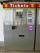In the ticket machine were together one hundred coins. They were only 20 and 50 cent coins. The sum total was 29 euros and 60 cents. How many were in ticket machine coins, and which type?
• Car rent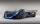Rental cars are paid a fixed daily fee plus 16 cents for each kilometer traveled. Samuel want to rent a car for a day and worked out that will pay 175 euros. When paying he got a 20% discount on the daily fee, the cost per kilometer is unchanged. Samuel t
• Divide money 2Ben and Dan had the same amount of money at the start. When Ben gave 300 to Dan, the ratio of Ben's money to Dan's money became 2:3. How much money did each have at first?The school received money from the sponsor. She used one-third to buy computers, half of the rest to adjust the gym. It still has 4000 euros left. How many euros did the sponsor donate to the school?
• Three workers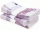The three workers received € 2,850 together for the work done. They divided them according to the time worked so that the first received 20% less than the second and the third € 50 more than the second. How much EUR did each worker receive?
• RepairingThree employees earned a total of € 469 for repairing the equipment. They split so that the first got 20% more than the second, and the third 15% more than the second. How many euros did everyone get?
• Four friendsFour friends shared the money. Vasek got 1/4 of the total amount. Tonda received 1/3 of the rest of the money, Joe got a half from the second residue and Jirka left 80. How much money get together?
• Salary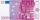Lawyer got to pay 840 Euros in banknotes of 20 and 50 Eur. Total got 18 banknotes. How many was which?
• Class 9.CProfessor collects money in the 9. C for a school trip. 2/3 of the collected amount was from girls and 1/4 from boys. The rest of 410 CZK went from the class fund. How much will the school trip cost in total?
• Warehouses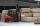To four warehouses is going cement in 25 kg bags. To first one third, to second quarter of the total. The third store got two thirds of the rest, and the last 310 tons came to fourth. How many cement is in all warehouses and how much got every one?
• Dividing moneyMilan divided 360 euros in the ratio 4: 5, Erik in the ratio 500: 625. How many euros did the individual parts of Milan and how many Erik?
• Soccer balls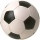Pupils in one class want to buy two soccer balls together. If each of them brings 12.50 euros, they will miss 100 euros; if each brings 16 euros, they will remain 12 euros. How many students are in the class?
• Paul earnedPaul earned 300 Kč in one hour, Václav 1/3 more than Paul. Václav worked 60 hours, which is 1/3 fewer hours than Paul worked. How many percent less earned Paul an hour than Václav? How many hours did Paul more than Václav? How much did Paul earn more than
• Three friends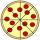Divide 570 euros to three friends so that first will get 50 euros less than the second and third twice more than the first. How many euros will get everyone?